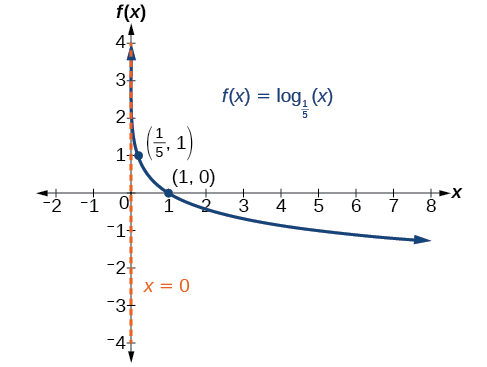# 6.4 Graphs of logarithmic functions  (Page 3/8)

 Page 3 / 8

Given a logarithmic function with the form $\text{\hspace{0.17em}}f\left(x\right)={\mathrm{log}}_{b}\left(x\right),$ graph the function.

1. Draw and label the vertical asymptote, $\text{\hspace{0.17em}}x=0.$
2. Plot the x- intercept, $\text{\hspace{0.17em}}\left(1,0\right).$
3. Plot the key point $\text{\hspace{0.17em}}\left(b,1\right).$
4. Draw a smooth curve through the points.
5. State the domain, $\text{\hspace{0.17em}}\left(0,\infty \right),$ the range, $\text{\hspace{0.17em}}\left(-\infty ,\infty \right),$ and the vertical asymptote, $\text{\hspace{0.17em}}x=0.$

## Graphing a logarithmic function with the form f ( x ) = log b ( x ).

Graph $\text{\hspace{0.17em}}f\left(x\right)={\mathrm{log}}_{5}\left(x\right).\text{\hspace{0.17em}}$ State the domain, range, and asymptote.

Before graphing, identify the behavior and key points for the graph.

• Since $\text{\hspace{0.17em}}b=5\text{\hspace{0.17em}}$ is greater than one, we know the function is increasing. The left tail of the graph will approach the vertical asymptote $\text{\hspace{0.17em}}x=0,$ and the right tail will increase slowly without bound.
• The x -intercept is $\text{\hspace{0.17em}}\left(1,0\right).$
• The key point $\text{\hspace{0.17em}}\left(5,1\right)\text{\hspace{0.17em}}$ is on the graph.
• We draw and label the asymptote, plot and label the points, and draw a smooth curve through the points (see [link] ).

The domain is $\text{\hspace{0.17em}}\left(0,\infty \right),$ the range is $\text{\hspace{0.17em}}\left(-\infty ,\infty \right),$ and the vertical asymptote is $\text{\hspace{0.17em}}x=0.$

Graph $\text{\hspace{0.17em}}f\left(x\right)={\mathrm{log}}_{\frac{1}{5}}\left(x\right).\text{\hspace{0.17em}}$ State the domain, range, and asymptote.The domain is $\text{\hspace{0.17em}}\left(0,\infty \right),$ the range is $\text{\hspace{0.17em}}\left(-\infty ,\infty \right),$ and the vertical asymptote is $\text{\hspace{0.17em}}x=0.$

## Graphing transformations of logarithmic functions

As we mentioned in the beginning of the section, transformations of logarithmic graphs behave similarly to those of other parent functions. We can shift, stretch, compress, and reflect the parent function $\text{\hspace{0.17em}}y={\mathrm{log}}_{b}\left(x\right)\text{\hspace{0.17em}}$ without loss of shape.

## Graphing a horizontal shift of f ( x ) = log b ( x )

When a constant $\text{\hspace{0.17em}}c\text{\hspace{0.17em}}$ is added to the input of the parent function $\text{\hspace{0.17em}}f\left(x\right)=lo{g}_{b}\left(x\right),$ the result is a horizontal shift     $\text{\hspace{0.17em}}c\text{\hspace{0.17em}}$ units in the opposite direction of the sign on $\text{\hspace{0.17em}}c.\text{\hspace{0.17em}}$ To visualize horizontal shifts, we can observe the general graph of the parent function $f\left(x\right)={\mathrm{log}}_{b}\left(x\right)\text{\hspace{0.17em}}$ and for $\text{\hspace{0.17em}}c>0\text{\hspace{0.17em}}$ alongside the shift left, $\text{\hspace{0.17em}}g\left(x\right)={\mathrm{log}}_{b}\left(x+c\right),$ and the shift right, $\text{\hspace{0.17em}}h\left(x\right)={\mathrm{log}}_{b}\left(x-c\right).$ See [link] .

## Horizontal shifts of the parent function y = log b ( x )

For any constant $\text{\hspace{0.17em}}c,$ the function $\text{\hspace{0.17em}}f\left(x\right)={\mathrm{log}}_{b}\left(x+c\right)$

• shifts the parent function $\text{\hspace{0.17em}}y={\mathrm{log}}_{b}\left(x\right)\text{\hspace{0.17em}}$ left $\text{\hspace{0.17em}}c\text{\hspace{0.17em}}$ units if $\text{\hspace{0.17em}}c>0.$
• shifts the parent function $\text{\hspace{0.17em}}y={\mathrm{log}}_{b}\left(x\right)\text{\hspace{0.17em}}$ right $\text{\hspace{0.17em}}c\text{\hspace{0.17em}}$ units if $\text{\hspace{0.17em}}c<0.$
• has the vertical asymptote $\text{\hspace{0.17em}}x=-c.$
• has domain $\text{\hspace{0.17em}}\left(-c,\infty \right).$
• has range $\text{\hspace{0.17em}}\left(-\infty ,\infty \right).$

Given a logarithmic function with the form $\text{\hspace{0.17em}}f\left(x\right)={\mathrm{log}}_{b}\left(x+c\right),$ graph the translation.

1. Identify the horizontal shift:
1. If $\text{\hspace{0.17em}}c>0,$ shift the graph of $\text{\hspace{0.17em}}f\left(x\right)={\mathrm{log}}_{b}\left(x\right)\text{\hspace{0.17em}}$ left $\text{\hspace{0.17em}}c\text{\hspace{0.17em}}$ units.
2. If $\text{\hspace{0.17em}}c<0,$ shift the graph of $\text{\hspace{0.17em}}f\left(x\right)={\mathrm{log}}_{b}\left(x\right)\text{\hspace{0.17em}}$ right $\text{\hspace{0.17em}}c\text{\hspace{0.17em}}$ units.
2. Draw the vertical asymptote $\text{\hspace{0.17em}}x=-c.$
3. Identify three key points from the parent function. Find new coordinates for the shifted functions by subtracting $\text{\hspace{0.17em}}c\text{\hspace{0.17em}}$ from the $\text{\hspace{0.17em}}x\text{\hspace{0.17em}}$ coordinate.
4. Label the three points.
5. The Domain is $\text{\hspace{0.17em}}\left(-c,\infty \right),$ the range is $\text{\hspace{0.17em}}\left(-\infty ,\infty \right),$ and the vertical asymptote is $\text{\hspace{0.17em}}x=-c.$

## Graphing a horizontal shift of the parent function y = log b ( x )

Sketch the horizontal shift $\text{\hspace{0.17em}}f\left(x\right)={\mathrm{log}}_{3}\left(x-2\right)\text{\hspace{0.17em}}$ alongside its parent function. Include the key points and asymptotes on the graph. State the domain, range, and asymptote.

Since the function is $\text{\hspace{0.17em}}f\left(x\right)={\mathrm{log}}_{3}\left(x-2\right),$ we notice $\text{\hspace{0.17em}}x+\left(-2\right)=x–2.$

Thus $\text{\hspace{0.17em}}c=-2,$ so $\text{\hspace{0.17em}}c<0.\text{\hspace{0.17em}}$ This means we will shift the function $\text{\hspace{0.17em}}f\left(x\right)={\mathrm{log}}_{3}\left(x\right)\text{\hspace{0.17em}}$ right 2 units.

The vertical asymptote is $\text{\hspace{0.17em}}x=-\left(-2\right)\text{\hspace{0.17em}}$ or $\text{\hspace{0.17em}}x=2.$

Consider the three key points from the parent function, $\text{\hspace{0.17em}}\left(\frac{1}{3},-1\right),$ $\left(1,0\right),$ and $\text{\hspace{0.17em}}\left(3,1\right).$

The new coordinates are found by adding 2 to the $\text{\hspace{0.17em}}x\text{\hspace{0.17em}}$ coordinates.

Label the points $\text{\hspace{0.17em}}\left(\frac{7}{3},-1\right),$ $\left(3,0\right),$ and $\text{\hspace{0.17em}}\left(5,1\right).$

The domain is $\text{\hspace{0.17em}}\left(2,\infty \right),$ the range is $\text{\hspace{0.17em}}\left(-\infty ,\infty \right),$ and the vertical asymptote is $\text{\hspace{0.17em}}x=2.$

More example of algebra and trigo
What is Indices
If one side only of a triangle is given is it possible to solve for the unkown two sides?
please I need help in maths
Okey tell me, what's your problem is?
Navin
the least possible degree ?
(1+cosA)(1-cosA)=sin^2A
good
Neha
why I'm sending you solved question
Mirza
Teach me abt the echelon method
Khamis
exact value of cos(π/3-π/4)
What is differentiation?
modul questions trigonometry
(1+cosA)(1-cosA)=sin^2A
BINCY
differentiate f(t)=1/4t to the power 4 +8
I need trigonometry,polynomial
ok
Augustine
Why is 7 on top
simplify cot x / csc x
👉🌹Solve🌻 Given that: cotx/cosx =cosx/sinx/cosx =1/sinx =cosecx Ans.
Vijay
what is the period of cos?
Patrick
simplify: cot x/csc x
Catherine
sorry i didnt realize you were actually asking someone else to put their question on here. i thought this was where i was supposed to.
Catherine
some to dereve formula for bulky density
kurash
Solve Given that: cotx/cosx =cosx/sinx/cosx =1/sinx =cosecx Ans.
Vijay
if tan alpha + beta is equal to sin x + Y then prove that X square + Y square - 2 I got hyperbole 2 Beta + 1 is equal to zero
questions
Thamarai
ok
AjAByByByBy Anindyo MukhopadhyayByBy